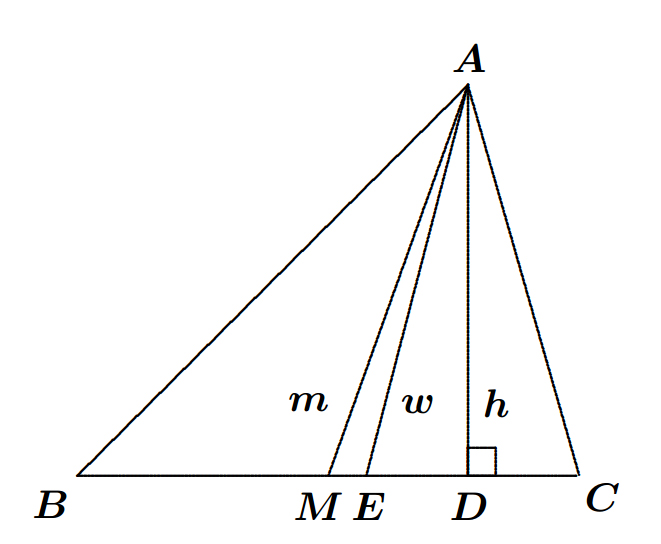# Now we're introducing "Limits" in Geometry!Let $m,w$ and $h$ be the lengths of the median, the angle bisector and the altitude, respectively, from the right-angled vertex $A$ of a triangle $ABC$ to the hypotenuse. Suppose that the side $c$ is fixed in length, while the side $b$ varies subject to $a>b \geq c$. Evaluate the value of the following correct upto three places of decimals:

$\large{\lim_{b \to c} \dfrac{m-h}{w-h}=\ ?}$

×